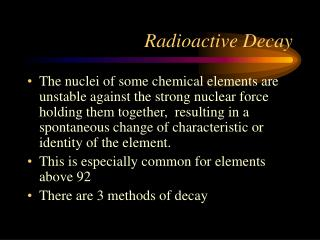DownloadDownload PresentationTélécharger la présentation- - - - - - - - - - - - - - - - - - - - - - - - - - - E N D - - - - - - - - - - - - - - - - - - - - - - - - - - -
##### Presentation Transcript

1. Radioactive Decay • The nuclei of some chemical elements are unstable against the strong nuclear force holding them together, resulting in a spontaneous change of characteristic or identity of the element. • This is especially common for elements above 92 • There are 3 methods of decay

2.  - decay  - decay  - decay A Helium nucleus seems to emerge from the unstable element An electron and neutrino emerge from the unstable element A photon emerges from the unstable element Decay Mechanisims

3.  - decay • Helium nucleus emerges from the unstable element. • 92 238U  90234Th + 24He + energy • electric repulsion becomes greater than the nuclear attraction/ contrast between short and long range forces. • Masses do not balance! …Why?

4.  - decay • An electron and a “neutrino” emerge from the unstable nucleus. • 614C 714N + - 10e + energy • Weak force - a “down quark” in a neutron changes into an “up quark” changing it into a proton. • Masses do not balance! …Why?

5.  - decay • Photon emerges from the unstable element • The element retains its identity • 1327 Al* 1327 Al +  + energy • nucleus is not changed but has an excess of energy - particles are agitated and farther away from each other. • Masses don’t balance!

6. Masses don’t balance! • Einstein - mass IS energy • E = mc2 • m is the mass difference between the parent nuclei and the daughters. The equation gives the energy released. Mass is converted into energy!

7. Decay Process • The fraction of atoms decaying in a time interval t is: • The value of depends on the nucleus • The unit of  is 1/seconds (per second) • N / N = - t

8. Decay Measurement In the LabMeasuring λ for an element: Villanovium • N / N = - t 2. Timer for Geiger Counter Vu 3. Count decay particles during time interval 1. How may atoms of Villanovium?

9. The Decay Equation • The fraction of atoms decaying in a time interval t is: • Using calculus on this equation, we get: N(t) - # at time t N0 - # at beginningt - elapsed timee = 2.71828… N / N = - t N(t) / N0 = e -t

10. Decay Equation – how it works N(t) / N0 = e -t • How does N(t) decrease with time?

11. ADD a fraction each time – compound interest N(t) / N0 = e +t

12. Decay Equation – how it works N(t) / N0 = e -t • Suppose 10% decays each second: 50%, half-life 

13. Half-Life – when ½ remains N(t) / N0 = e -t • Half-life [ t½ ] when 10% decays in a second --- about 6.4 seconds NEVER REACHES 0 Seconds

14. If you know  , you can find t1/2 • Suppose that 50% is left, then: • Since we know  we can solve for “t1/2”. We call that time the half-life – how long it takes (in seconds) for ½ of the sample to decay. N(t) / N0 = e -t = 0.5

15. Isotope Half-Life Cesium 137 30 Years Chromium 51 27.7 Days Cobalt 57 271.7 Days Cobalt 60 5.26 Years Copper 64 12.7 Hours Fluorine 18 109.7 Min Gadolinium 153 242 Days Gallium 67 3.26 Days Gold 198 2.7 Days Indium 111 2.81 Days Iodine 123 13.2 Hours Iodine 125 59.6 Days Iodine 131 8.02 Days Isotope Half-Life Iridium 192 73.83 Days Molybdenum 99 66 Hours Phosphorus 32 14.29 Days Samarium 153 46.7 Hours Selenium 75 119.8 Days Strontium 89 50.5 Days Technetium 99m 6.01 Hours Thallium 201 3.04 Days Xenon 133 5.2 Days Yttrium 90 64 Hours Half-life examples